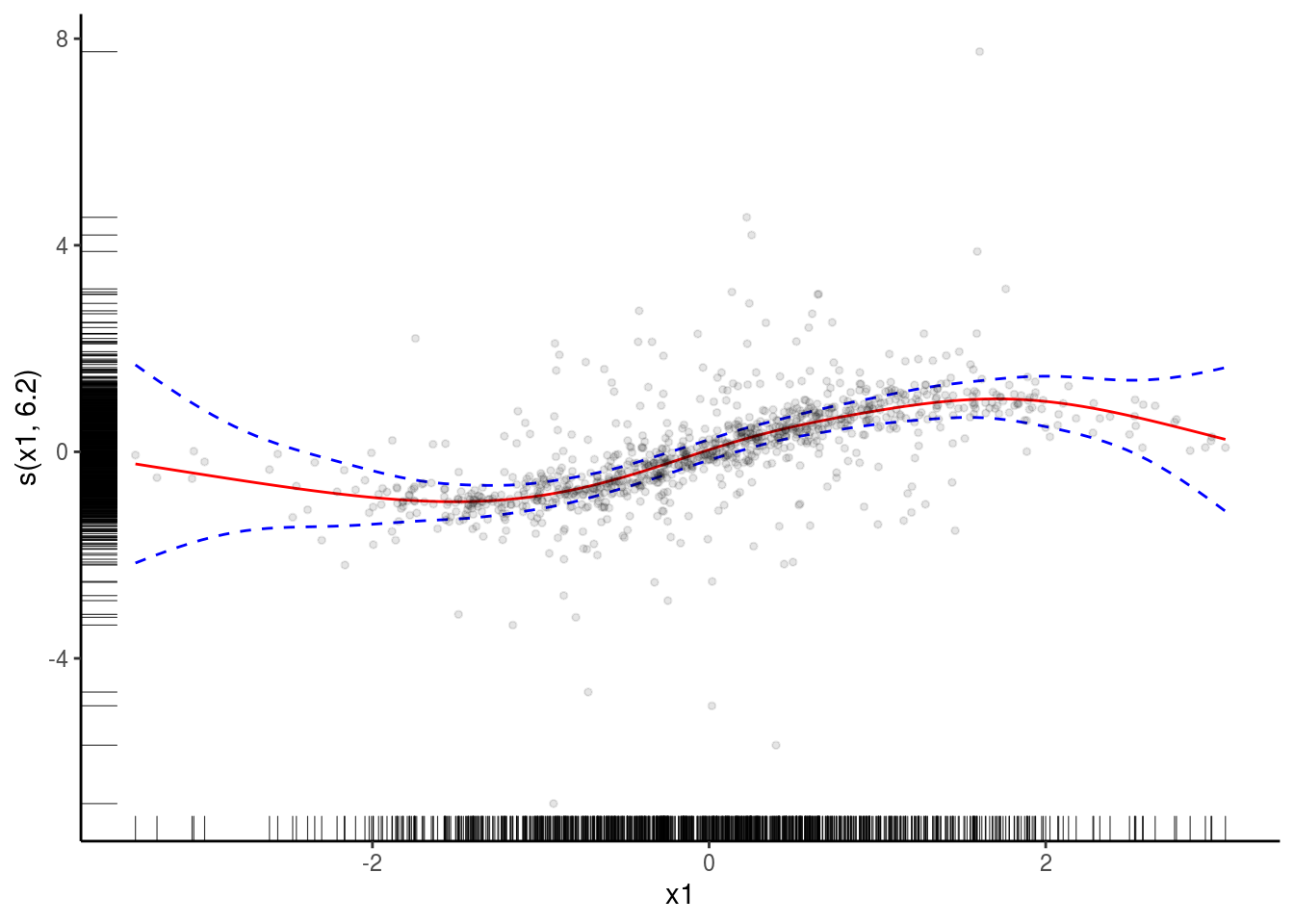# 1 mgcViz basics

The `mgcViz` R package (Fasiolo et al, 2018) offers visual tools for Generalized Additive Models (GAMs). The visualizations provided by `mgcViz` differs from those implemented in `mgcv`, in that most of the plots are based on `ggplot2`’s powerful layering system. This has been implemented by wrapping several `ggplot2` layers and integrating them with computations specific to GAM models. Further, `mgcViz` uses binning and/or sub-sampling to produce plots that can scale to large datasets (\(n \approx 10^7\)), and offers a variety of new methods for visual model checking/selection.

This document introduces the following categories of visualizations:

1. smooth and parametric effect plots: layered plots based on `ggplot2` and interactive 3d visualizations based on the `rgl` library;

2. model checks: interactive QQ-plots, traditional residuals plots and layered residuals checks along one or two covariates;

3. special plots: differences-between-smooths plots in 1 or 2D and plotting slices of multidimensional smooth effects.

## 1.1 Layered smooth effect plots

Here we describe effect-specific plotting methods and then we move to the `plot.gamViz` function, which wraps several effect plots together.

### 1.1.1 Effect-specific plots

``````library(mgcViz)
n  <- 1e3
dat <- data.frame("x1" = rnorm(n), "x2" = rnorm(n), "x3" = rnorm(n))
dat\$y <- with(dat, sin(x1) + 0.5*x2^2 + 0.2*x3 + pmax(x2, 0.2) * rnorm(n))
b <- gam(y ~ s(x1) + s(x2) + x3, data = dat, method = "REML")``````

Now we convert the fitted object to the `gamViz` class. Doing this allows us to use the tools offered by `mgcViz` and to save quite a lot of time when producing multiple plots using the same fitted GAM model.

``b <- getViz(b)``

We extract the first smooth component using the `sm` function and we plot it. The resulting `o` object contains, among other things, a `ggplot` object. This allows us to add several visual layers.

``````o <- plot( sm(b, 1) )
o + l_fitLine(colour = "red") + l_rug(mapping = aes(x=x, y=y), alpha = 0.8) +
l_ciLine(mul = 5, colour = "blue", linetype = 2) +
l_points(shape = 19, size = 1, alpha = 0.1) + theme_classic()``````We added the fitted smooth effect, rugs on the x and y axes, confidence lines at 5 standard deviations, partial residual points and we changed the plotting theme to `ggplot2::theme_classic`. Functions such as `l_fitLine` or `l_rug` are effect-specific layers. To see all the layers available for each effect plot we do:

``listLayers(o)``
``````##  "l_ciLine"  "l_ciPoly"  "l_dens2D"  "l_fitDens" "l_fitLine" "l_points"
##  "l_rug"     "l_simLine"``````

Similar methods exist for 2D smooth effect plots, for instance if we fit:

``````b <- gam(y ~ s(x1, x2) + x3, data = dat, method = "REML")
b <- getViz(b)``````

we can do

``plot(sm(b, 1)) + l_fitRaster() + l_fitContour() + l_points()``